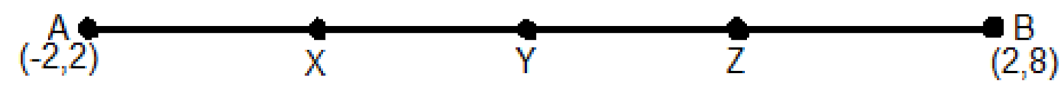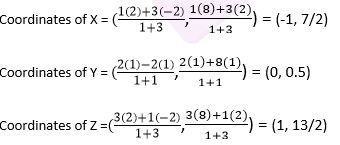Newbie

# Find the coordinates of the points which divide the line segment joining A (- 2, 2) and B (2, 8) into four equal parts.Q9

• 1

What is the best solution of coordinate geometry , how i solve this question easily  .Find the coordinates of the points which divide the line segment joining A (- 2, 2) and B (2, 8) into four equal parts.

Share